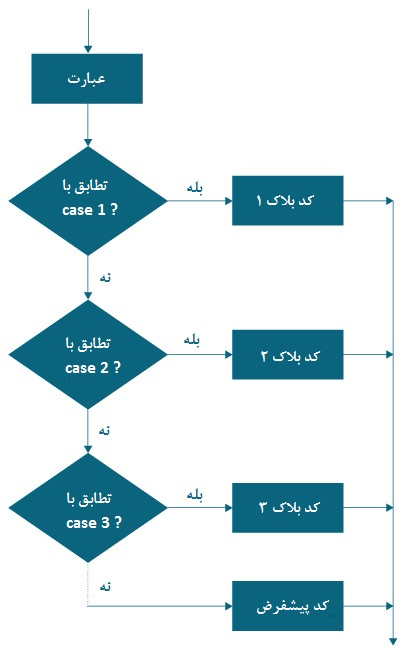# blog posts# In this tutorial you will learn to use the switch command to control the flow of your program. In Java, the if..else..if ladder executes code from many blocks.

The switch command can replace long ladders that make the code legible.

The syntax of the switch command is as follows:

switch (variable / expression) {

case value1:

// statements

break;

case value2:

// statements

break;

.. ..…

.. ..…

default:

// statements

}

The switch command evaluates the expression (mostly variable) and compares it with the values ​​(can be an expression) of each label.

The switch command executes all commands of the matching label.

Suppose the variable / expression is equal to value2. In this case, all commands related to this adaptive case are executed.

Note: Use the phrase break. This terminates the execution of the switch statement. Break statements are important because if they are not used, all commands after the matching tag will be executed in sequence until the end of the switch statement.

## Flowchart command switchIt should also be noted that the switch command in Java only works with the following:

• Initial data types: byte, short, char and int
• Types (enum (enum Java
• String class
• Character, Byte, Short and Integer classes.

Example 1: Switch command in Java

1. class Day {
2. public static void main (String [] args) {
3. int week = 4;
4. String day;
5. switch (week) {
6. case 1:
7. day = “Sunday”;
8. break;
9. case 2:
10. day = “Monday”;
11. break;
12. case 3:
13. day = “Tuesday”;
14. break;
15. case 4:
16. day = “Wednesday”;
17. break;
18. case 5:
19. day = “Thursday”;
20. break;
21. case 6:
22. day = “Friday”;
23. break;
24. case 7:
25. day = “Saturday”;
26. break;
27. default:
28. day = “Invalid day”;
29. break;
30. }
31. System.out.println (day);
32. }
33. }

Output

Wednesday

Example 2: Switch command in Java

The following program receives three inputs from the user: an operator and 2 numbers. Performs calculations based on numbers and operators entered. The result is then displayed.

We used the scanner object to get input from the user.

1. import java.util.Scanner;
2. class Calculator {
3. public static void main (String [] args) {
4. char operator;
5. Double number1, number2, result;
6. Scanner scanner = new Scanner (System.in);
7. System.out.print (“Enter operator (either +, -, * or /):”);
8. operator = scanner.next (). charAt (0);
9. System.out.print (“Enter number1 and number2 respectively:”);
10. number1 = scanner.nextDouble ();
11. number2 = scanner.nextDouble ();
12. switch (operator) {
13. case ‘+’:
14. result = number1 + number2;
15. System.out.print (number1 + “+” + number2 + ”=” + result);
16. break;
17. case ‘-‘:
18. result = number1 – number2;
19. System.out.print (number1 + “-” + number2 + ”=” + result);
20. break;
21. case ‘*’:
22. result = number1 * number2;
23. System.out.print (number1 + “*” + number2 + ”=” + result);
24. break;
25. case ‘/’:
26. result = number1 / number2;
27. System.out.print (number1 + “/” + number2 + ”=” + result);
28. break;
29. default:
30. System.out.println (“Invalid operator!”);
31. break;
32. }
33. }
34. }

Output

Enter operator (either +, -, * or /): *

Enter number1 and number2 respectively: 1.4

-5.3

1.4 * -5.3 = -7.419999999999999